In mathematics, the problem of solid of revolution is sometimes asked. It involves calculating the volume and surface area of a plane figure after one rotation.

How to solve such a solid of revolution problem? For a complex solid of revolution, we need to learn high school mathematics integration to be able to calculate them. On the other hand, simple figures such as triangles and squares in solid of revolution can be solved with simple math knowledge.

However, when solving solid of revolution problems, it is necessary to understand what the shape of the solid of revolution will look like. Also, there are advanced problems that combine various shapes. For this reason, the problems in a solid of revolution are very challenging.

In this article, we will explain the basics of a solid of revolution in mathematics and how to solve the problems.

## A Shape Created by Rotating Around an Axis Is a Solid of Revolution

Space figures include prisms, cylinders, pyramids, cones, and spheres. However, there is a problem that must be considered as a space figure, even though it is a plane figure. It is the solid of revolution.

A solid of revolution refers to a figure that is completed by a single rotation of an axis, as shown below.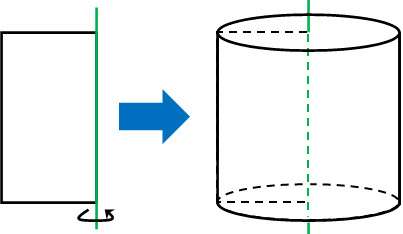This is the solid of revolution. We have to create a space figure from a plane figure. You have to imagine in your mind what kind of figure will be completed.

Then we calculate the volume or surface area of the solid of revolution. For example, what would be the volume and surface area of the following solid of revolution?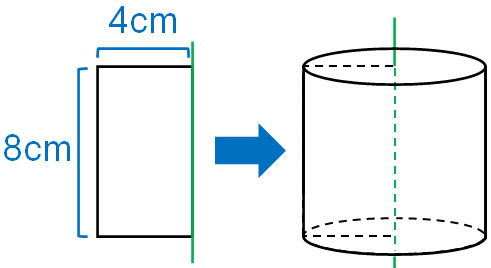The volume of the cylinder can be calculated by multiplying the base area by the height. Therefore, the volume of this solid of revolution is $128π$ cm3.

• $4×4×π×8=128π$

The surface area, on the other hand, can be calculated by adding the bottom areas and the side area. The sum of the base area is as follows.

• $4×4×π×2=32π$

Also, the side area of the cylinder is as follows.

• $8×π×8=64π$

Therefore, the surface area of the solid of revolution is $32π+64π=96π$, and the answer is $96π$ cm2. After rotating the plane figure in a straight line, calculate the volume and surface area.

### The Base of a Solid of Revolution Will Always Be a Circle

Solids of revolution have one thing in common: they are always circular. In other words, they will never be prismatic or pyramidal space figures. A solid of revolution always have a curve.

As an example, here are the triangular and semicircular solids of revolution.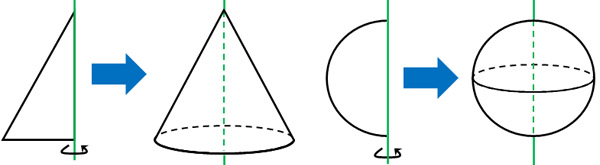Earlier, we explained that a rectangular solid of revolution becomes a cylinder. On the other hand, a triangular solid of revolution becomes a cone. A semicircle solid of revolution becomes a sphere.

When you revolve a triangle or a rectangle, its base will always be a circle. On the other hand, there is no base for a semicircle solid of revolution. It is the same, however, that the solid of revolution has a curved figure.

### Use Formulas for Cylinders, Cones, and Spheres

In this way, we can imagine a three-dimensional object in terms of space figures. Then, use the formulas to solve the problems.

We already know how to calculate cylinders. Multiplying the base area by the height, we can get the cylinder volume. For a cone, we can also calculate it by multiplying the volume of the cylinder by 1/3.

• Cylinder volume = Base area × Height
• Cone volume = Base area × Height × 1/3

The surface area, on the other hand, is calculated by summing up the base area and side area.

In the case of spheres, use the formulas to find the volume and surface area. Although the calculation of spheres is infrequent, if you do not remember the formula, you will not be able to solve the problem. If you want to solve the sphere problem, try to remember the following formulas.

-The Formula for the Sphere Volume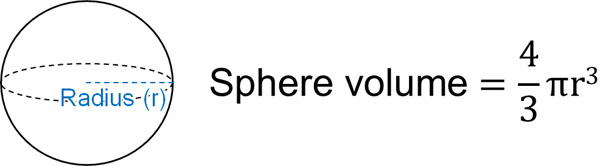If we know the radius, we can calculate the volume of the sphere by substituting the number into the formula.

-The Formula for the Sphere Surface Area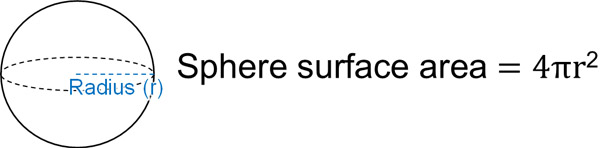Similarly, if we know the radius, we can substitute it into the surface area formula. We can calculate the surface area of a solid of revolution.

## The Advanced Problem Is Combining Figures.

One of the advanced problems for solids of revolution is the combination of shapes. If there are several types of figures, the shape of the solid of revolution becomes more complicated.

For example, let’s consider the following solid of revolution.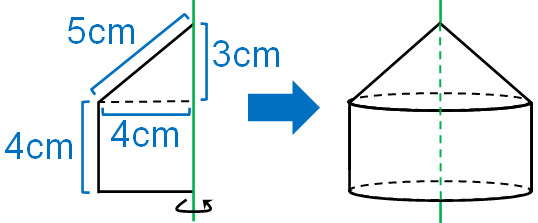This figure is a mixture of a triangle and a square. In this type of solid of revolution, a cone and a cylinder are mixed together. In such cases, separate the figures and calculate the volume and surface area.

-Calculation of Volume

Let’s calculate the volume of a cone and a cylinder, respectively. The volume of the cone is as follows.

• $4×4×π×3×\displaystyle\frac{1}{3}=16π$

And the volume of the cylinder is as follows.

• $4×4×π×4=64π$

By adding the volumes of the cone and the cylinder, we find that the answer is $16π+64π=80π$ cm3.

-Calculation of Surface Area

In calculating surface area, we need to think about the net. In other words, we need to think about the space figure and then convert it to a plane. The result is as follows.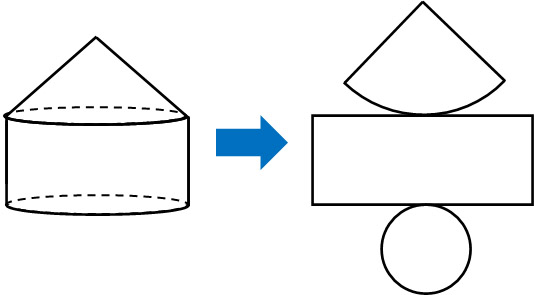After calculating the area of each, make sure to add them up. The result is as follows.

• Area of a sector: $5×4×π=20π$
• Area of a rectangle: $8×π×4=32π$
• Area of a circle: $4×4×π=16π$

For reference, we use the following formula for the sector area.

• Side area of a cone = Generatrix × Radius of the base × $π$

The answer for the surface area of the solid is $68π$ cm2 by adding these areas.

• $20π+32π+16π=68π$

### The Shape of a Solid of Revolution Whose Plane Is Away from Its Axis

Incidentally, there are some cases where the plane is away from the axis when making a solid of revolution. In that case, a solid of revolution with a hollow space is created.

We have already explained that a rectangular solid of revolution becomes a cylinder. On the other hand, if the rectangle is away from the line of rotation, the solid of revolution will be a donut shape as shown below.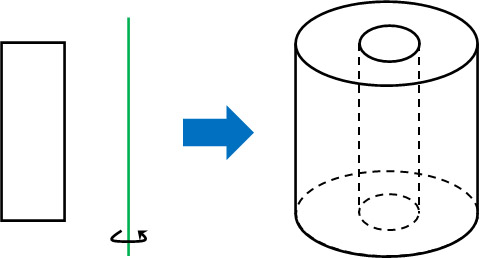When calculating the volume or surface area of this figure, we have to consider the two cylinders. In any case, consider that a hollow can be created if the plane is away from the axis of rotation.

## Exercise: Volume and Surface Area of a Solid of Revolution

Q1: For the following figure, calculate the volume and surface area of the figure formed by making one rotation around a straight line. Let pi be $π$.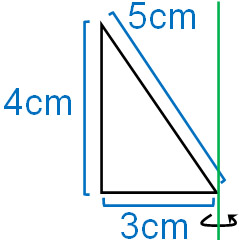The solid of revolution of this figure is as follows.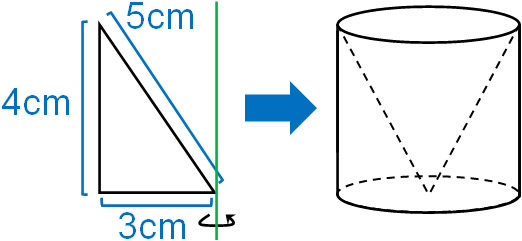In calculating solids of revolution, we frequently have to calculate a figure that combines a cone and a cylinder. In this figure, there is a cone inside a cylinder. Therefore, let’s calculate the cylinder and cone separately.

-Calculation of Volume

If we subtract a cone from a cylinder, we can get the volume. The volume of the cylinder is as follows.

• $3×3×π×4=36π$

On the other hand, the volume of the cone is as follows.

• $3×3×π×4×\displaystyle\frac{1}{3}=12π$

Therefore, the volume of the solid is $24π$ cm3.

• $36π-12π=24π$

-Calculation of Surface Area

The calculation method is the same as that of the triangle and rectangle solid of revolution. If we consider the net, we can see three shapes: a sector, a rectangle, and a circle. So, let’s calculate the area of each.

• Area of a sector: $5×3×π=15π$
• Area of a rectangle: $6×π×4=24π$
• Area of a circle: $3×3×π=9π$

If we add the areas of each, we get $48π$ cm2.

• $15π+24π+9π=48π$

## Learning the Basics of Solids of Revolution in Space Figures

In Space Figures, we learn about the concept of solids of revolution. A solid of revolution is a space figure created by rotating a plane around an axis.

In the case of a complex solid of revolution, we need to use integrals in high school mathematics to solve the problem. On the other hand, simple solids of revolution, such as triangles and squares, can be solved without the use of integrals. So, use the formulas for cones, cylinders, and spheres to do the calculations.

In advanced problems, multiple figures will be combined. Or, the figures may be separated from the axis. However, the basic idea is the same.

Among the space figures, the problem of finding the volume and surface area of a solid of revolution is more difficult. Try to imagine what kind of solid of revolution you can make and calculate the volume and surface area.